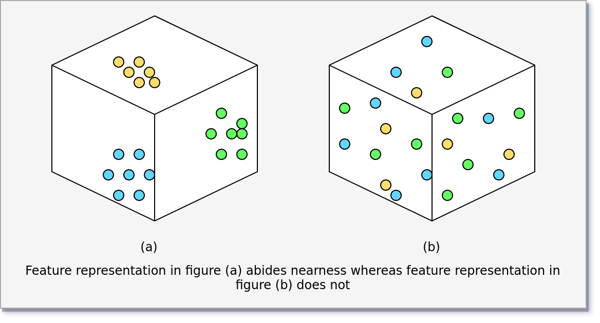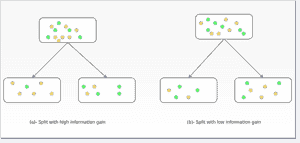Related Tags

machine learning

# What is an inductive bias?

Esha Fatima

### Overview

Machine learning models have evolved and gained much of their appraisal as they have refined the way that predictive models were built earlier. AI based systems that lay basis on rule-based models required extensive domain knowledge and were yet unable to capture all possible scenarios of a decisive space.

### How is training different?

Machine learning algorithms attempt to extrapolate and figure out patterns in instances of data that are provided to the system. Each instance in a dataset represents the type of data that we would ideally like our system to derive a relation within. By collecting numerous sample points, the algorithm evolves and tunes its parameters to neatly draw a correlation and predict the outcome when provided with a test point.

At this point, we imply certain assumptions about the nature of our training dataset in order to use the best possible machine learning algorithm. In other words, each machine learning model operates on a finely defined set of assumptions or inductive biases that are expected to be reflected in the data points that it attempts to model from.

### Nearest neighbors in k-NN

k-NN algorithm assumes that data points that are closelyCloseness is measured by calculating the distance between the respective features of the sample points via a suitable distance metric that could include Euclidean or Manhattan distance. situated in the feature space would cluster and exhibit the same predicted class. Accordingly, the algorithm relies heavily on this assumption to predict the class. Hence, it implies that the dataset should be such that closely related points fall under the same class.### Low depth in decision trees

Decision trees attempt to model the predictive class of an input instance by assessing the information gain associated with each possible class split. Hence, splits that result in high information gain is preferred over splits with low information gain. Under the hood, the algorithm tries to find the shortest possible classification route by preferring high information gain splits and consequently a low tree depth.### Linearity in regression

Linear regression attempts to model the predictive outcome via linear segregation in the feature space. For instance, the univariate linear regression model attempts to fit the best possible line in the graph representing the feature and the corresponding outcome. Similarly, multivariate linear regression attempts to form this hypothesis-based model by producing the best fitting plane. Hence, it is imperative for the data set to exhibit linear growth patterns so as to best benefit from linear regression. An example of a linear pattern could be the weight of a person with respect to their height. However, the area of circular ground with respect to its radius would be simply non-linear and not apt for linear regression.

### Conditional independence in Naive Bayes Classifiers

In Naive Bayes classification, the input variable in the feature space is assumed to be independent of every other input variable. An example of such an assumption is made in that of sentiment analysis in Natural Language ProcessingIt is the usage of machine learning to interpret and analyze written text corpora. In other words, the probability of each word occurring in a sentence is assumed to be the same regardless of what its preceding word is. This model of independence works fairly well in the case of language processing but fails miserably in cases where the input variables are significantly dependent on each other. For instance, in using SAT score and language skills to predict the university acceptance rate of a student, it would be flawed to say that SAT score is independent of language skills or vice versa. Hence, in such representations, the underlying premise of independence in the Naive Bayes classification fails.

### Why is inductive bias important?

It can be derived that it is essential to be aware of the inductive biases when tackling a machine learning based problem. Not every problem can be accurately represented via a single model due to differences in the semantics of data. Practically, a k-NN model would fail if the data set consists of random, spread-out points where data instances close to each other still do not fall under the same output class.

Consequently, it becomes an important matter of question as to what inductive biases a particular model possesses and whether or not our data fits within those constraints.

RELATED TAGS

machine learning

CONTRIBUTOR

Esha Fatima
RELATED COURSES

View all Courses

Keep Exploring

Learn in-demand tech skills in half the time